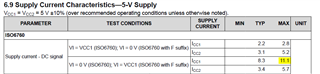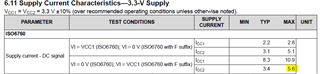If you have a related question, please click the "Ask a related question" button in the top right corner. The newly created question will be automatically linked to this question.

# ISO6760-Q1: Supply Current

Part Number: ISO6760-Q1

I am using device - ISO6760QDWRQ1 and wanted to know the supply currents (ICC1 & ICC2) when my VCC1=5V and VCC2=3.3V. I have considered below supply currents as maximum possible supply currents.

Is my understanding correct?Thanks,

Ritu

• Hello Ritu,

Thank you for your question. Yes, your understanding is correct. When VCC1=5V, the maximum possible supply current for ICC1 is 11.1 mA, and when VCC2=3.3V, the maximum possible supply current for ICC2 is 5.6 mA. This is assuming that you are using a DC signal. If you are using an AC signal (switching square wave), you can find maximum supply current values in the same table under the "AC signal" parameter section.

Regards,

Darrah